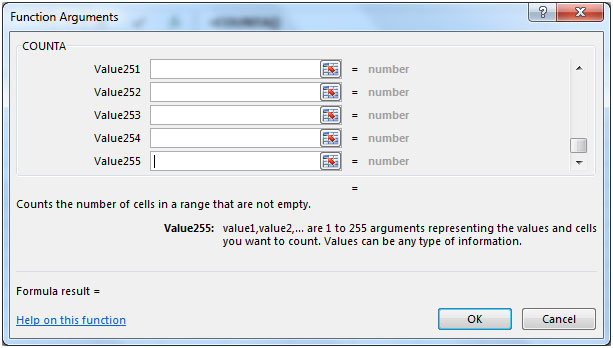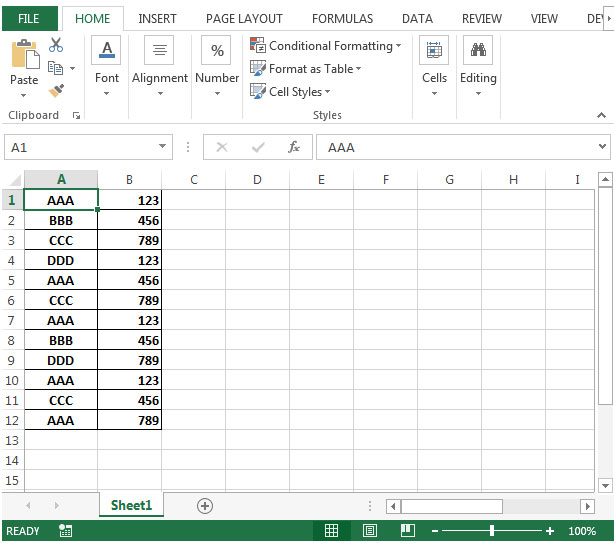Get the Row number of the last non blank cell in a column in Microsoft Excel

In this article, we will learn how to get last row number of the last non blank cell in a column in Microsoft Excel.

Consider a scenario in which you want to find the last non blank cell in a column.

Using a combination of three functions including ROW, COUNTA, and OFFSSET, you can devise an excel formula for last row which will find out the cell number of the last non blank cell in a column.

ROW: Returns the row number of a reference.

Syntax: =ROW(reference)

Reference: It is a reference to a cell or range of cells.

COUNTA: Counts the number of cells in a range that is not empty.

Syntax: =COUNTA(value1,value2,...)

value1: The first argument representing the values that you want to count.

value2: This is optional. It represents the values that you want to count

There can be maximum 255 arguments. Refer below screenshot:OFFSET: Returns a reference to a range that is a given number of rows and columns from a given reference.

Syntax: =OFFSET(reference,rows,cols,height,width)

reference: It is a reference to a cell or range of cells from which the OFFSET will be applied.

rows: The number of rows up or down that you want to apply as the offset to the range.

cols: The number of columns left or right that you want to apply as the offset to the range.

height: This is optional. It is the number of rows that you want the returned reference to be.

width: This is optional. It is the number of columns that you want the returned reference to be.

Let us take an example:

• In column A & B, we have some codes• We want to find out the last non blank cells. The formula in cell D2 would be =ROW(OFFSET(A1,COUNTA(A:A)-1,0))
• Cell A1 is taken as reference in Offset function
• Count A will be the (rows) second argument of Offset function & will return the total number of rows contained in column A.
• 0 is taken as cols the third argument. Formula will check for column A.
• The value returned by Offset & Count A will be used by Row to calculate the last non blank cell.

1.M Blasco's worked for me
Sid Le SLoth did not work at all, the formula had an error in it.

2.This formula, as already mentioned, is flawed. David / M Blasco's answer didn't work for me (Excel 2016). I found this formula at ExtendOffice.com (How to return the row number of first or last non blank cell in worksheet?):

=SUMPRODUCT(MAX((A:A"")*ROW(A:A)))

Don't ask me how it works!

3.David's answer almost worked for me, but I had to modify it a little (note: this formula won't work in the same column you are inspecting):

=MAX(IF(ISBLANK(A:A),0,ROW(A:A)))

4.Absolutely correct to Igor comments. Why do need all this if we can do it with COUNTA(A:A) ?

How do I know the last row if I have empty cells in column A?

•MAX(ARRAYFORMULA(IFERROR(IF(ISBLANK(A:A),0,ROW(A:A)))))

•There may be intervening blank cells. Counta will not be accurate.

5.Overcomplicated.
This would not work if there are a few empty cells in column A. Yet if this is not important, why not just count the number of non-blank cells. =COUNTA(A:A) will give the same result.

Terms and Conditions of use

The applications/code on this site are distributed as is and without warranties or liability. In no event shall the owner of the copyrights, or the authors of the applications/code be liable for any loss of profit, any problems or any damage resulting from the use or evaluation of the applications/code.